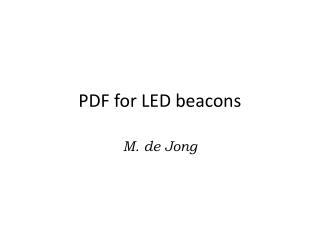DownloadDownload PresentationPDF for LED beacons

# PDF for LED beacons

Télécharger la présentation## PDF for LED beacons

- - - - - - - - - - - - - - - - - - - - - - - - - - - E N D - - - - - - - - - - - - - - - - - - - - - - - - - - -
##### Presentation Transcript

1. PDF for LED beacons M. de Jong

2. Probability Density Function • What? • The PDFis a function that describes the relative likelihood of a photon from a LED to be detected at a given arrival time. • Why? • A fit of the PDFto LED dataallows for a direct determination of the properties of the medium.

3. Topology z’ z y’ qr fr x’ PMT D 4D-bin q0 [D][cosq0][qr][fr] (0,0,0) LED

4. Input • Light source characteristics • i.e: Valencia? • Light propagation model • absorption • scattering • Rayleigh • Mie • Implementation¶ • single light scattering approximation ¶ ANTARES-SOFT-2011-004

5. Output • PDF as a function of arrival time for a given • distanceD • direction cosine cosq0 • orientation PMT qr, fr • Implementation • PDF = f(ls, labs, a, b, ...; t ) • where = f(a, b, ...)

6. Measurements • absorption • labs(l) • scattering • Rayleigh • ls(l) ( is known from theory) • Mie • ls(l) and = f(a, b, ...)?